# Littlewood problem

(diff) ← Older revision | Latest revision (diff) | Newer revision → (diff)

The Littlewood problem for compatible Diophantine approximations is the question of the existence, for any real numbers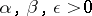, of a natural numbersuch that, where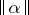is the distance fromto the nearest integer. In certain cases, for example for rationaland, and for numbersandone of which can be represented by a continued fraction with non-negative elements, the Littlewood problem has an affirmative answer.

The Littlewood problem for integrals is the problem whether for an arbitrary increasing sequenceof natural numbers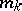,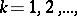one has(*)

with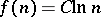, where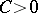is an absolute constant and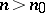. The following estimates have been obtained: either weaker estimates in comparison with (*) for arbitrary sequences, or estimates close to (*) or even coinciding with this estimate, but for special sequences.

The Littlewood problems were stated by J.E. Littlewood (see ).

How to Cite This Entry:
Littlewood problem. Encyclopedia of Mathematics. URL: http://encyclopediaofmath.org/index.php?title=Littlewood_problem&oldid=15076
This article was adapted from an original article by B.M. Bredikhin (originator), which appeared in Encyclopedia of Mathematics - ISBN 1402006098. See original article